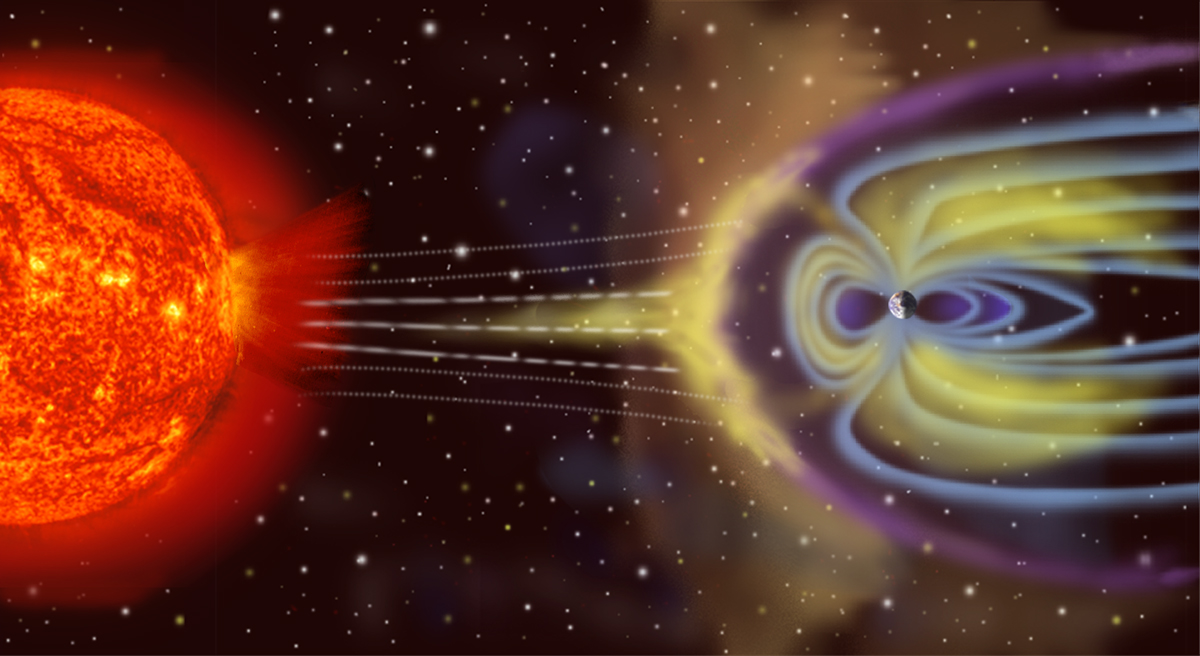# Crystals, Royal Rife and Maxwell’s equations

Royal Rife was famous for inventing a machine that could defeat cancer using Maxwell’s equations and crystals. Sadly for humanity, it was about that time of formation and rise to prominence of the American Medical Association (AMA) and the Federal Drug Administration (FDA), both biology based.

The scientific breakthrough work, to match pathogens to frequency, was proven by Royal Rife but his science was terminated and the man himself character-assassinated and run out of the country.

Crystals and Maxwell’s equations are in the news again, and a similar invention for curing cancer. A Silicon Valley start-up company called Theranos and a founder, woman by the name of Elizabeth Holmes is the next Royal Rife to be ended.

The reason I mention Maxwell’s equations is in the title is because he’s the first scientist to observe, measure and calculate the math involved in wave dynamics, here’s how it happens to be in the news and views.

Maxwell’s equations are a set of coupled partial differential equations that, together with the Lorentz force law, form the foundation of classical electromagnetism, classical optics, and electric circuits. The equations provide a mathematical model for electric, optical, and radio technologies, such as power generation, electric motors, wireless communication, lenses, radar etc.

Maxwell’s equations describe how electric and magnetic fields are generated by charges, currents, and changes of the fields. An important consequence of the equations is that they demonstrate how fluctuating electric and magnetic fields propagate at a constant speed (c) in a vacuum.

Known as electromagnetic radiation, these waves may occur at various wavelengths to produce a spectrum of light from radio waves to γ-rays. The equations are named after the physicist and mathematician James Clerk Maxwell, who between 1861 and 1862 published an early form of the equations that included the Lorentz force law. Maxwell first used the equations to propose that light is an electromagnetic phenomenon.

The equations have two major variants. The microscopic Maxwell equations have universal applicability but are unwieldy for common calculations. They relate the electric and magnetic fields to total charge and total current, including the complicated charges and currents in materials at the atomic scale.

The “macroscopic” Maxwell equations define two new auxiliary fields that describe the large-scale behaviour of matter without having to consider atomic scale charges and quantum phenomena like spins. However, their use requires experimentally determined parameters for a phenomenological description of the electromagnetic response of materials.In a geomagnetic storm, a surge in the flux of charged particles temporarily alters Earth’s magnetic field, which induces electric fields in Earth’s atmosphere, thus causing surges in electrical power grids. (Not to scale.)

The term “Maxwell’s equations” is often also used for equivalent alternative formulations. Versions of Maxwell’s equations based on the electric and magnetic potentials are preferred for explicitly solving the equations as a boundary value problem, analytical mechanics, or for use in quantum mechanics.

The covariant formulation (on spacetime rather than space and time separately) makes the compatibility of Maxwell’s equations with special relativity manifest. Maxwell’s equations in curved spacetime, commonly used in high energy and gravitational physics, are compatible with general relativity.

In fact, Einstein developed special and general relativity to accommodate the invariant speed of light, a consequence of Maxwell’s equations, with the principle that only relative movement has physical consequences.

Since the mid-20th century, it has been understood that Maxwell’s equations are not exact, but a classical limit of the fundamental theory of quantum electrodynamics.

Crystals Photo credit: Yagan Kiely on Visual Hunt / CC BY-SA